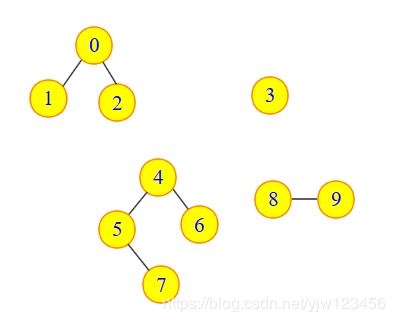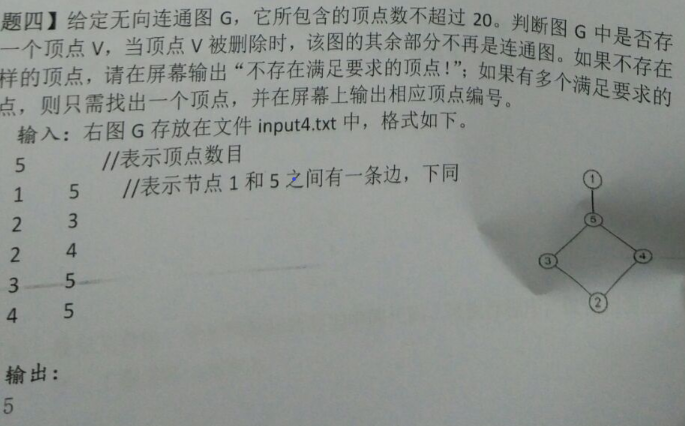• python networkx 没有函数可以实现 判断一个无向图中两个结点是否连通net python
• 有n个顶点，编号为1~n，用dfs遍历一遍邻接矩阵，若遍历到的顶点个数等于n，则证明改无向图一个连通图 #include<bits/stdc++.h> using namespace std; const int maxn=1005; bool vis[maxn]; vector<int...
 有n个顶点，编号为1~n，用dfs遍历一遍邻接矩阵，若遍历到的顶点个数等于n，则证明改无向图是一个连通图
#include<bits/stdc++.h>
using namespace std;
const int maxn=1005;
bool vis[maxn];
vector<int>G[maxn];
int n,m,k,number=0;
void dfs(int tmp) {
vis[tmp]=1;
cnt++;
for(int i=0; i<G[tmp].size(); i++) {
if(!vis[G[tmp][i]]) {
dfs(G[tmp][i]);
}
}
}
int main(){
int a,b;
cin>>n>>m;
while(m--){
cin>>a>>b;
G[a].push_back(b);
G[b].push_back(a);
}
dfs(1);
if(number==n) cout<<"YES"<<endl;
else cout<<"NO"<<endl;
return 0;
}

展开全文• (注意：判断一个无向图是否连通) 求一个无向图连通分量。 输入描述 第一行输入无向图的顶点数和边的条数，以空格隔开 第二行输入每个顶点的数据，中间没有空格 第三行输入每条边，每条边的格式为i j，中间空格，...
问题描述
已知无向图的顶点为字符型，要求采用邻接矩阵表示，图中顶点序号按字符顺序排列，从键盘输入图中顶点的个数、边的条数、顶点的信息和边的组成等。(注意：判断一个无向图是否连通) 求一个无向图的连通分量。 输入描述 第一行输入无向图的顶点数和边的条数，以空格隔开 第二行输入每个顶点的数据，中间没有空格 第三行输入每条边，每条边的格式为i j，中间有空格，所有边占一行 输出描述 输出该无向图的连通分量，占一行 输入样例 5 5 ABCDE 0 1 0 4 1 2 2 3 3 4 输出样例 1
问题分析
首先要清楚什么是无向图，在图的结构中每两个点之间最多只有一条线，这条线即代表了由a指向b，也代表了由b指向a，连通分量的概念：在上图中连通分量就是4.所以说连通分量就是在图中非连通子图的数量。
代码
#include<iostream>
using namespace std;
const int MaxSize = 20;
struct EdgeNode						//保存边表结点其中有下一个结点的下表和指向下一个结点的指针
{
EdgeNode *next;					//下一结点
};

struct VertexNode
{
char vertex;					//保存顶点
EdgeNode *firstEdge;			//指针域，指向下一个结点
};

class AlGraph
{
public:
AlGraph();
~AlGraph();
void BFTraverse(int v);			//广度优先遍历
int visited[MaxSize];
int EdgeNum,VertexNum; 			//保存顶点和边的个数
private:

};

AlGraph::AlGraph()
{
int x, y;								//用来保存边
EdgeNode *s = NULL;
cin >> VertexNum >> EdgeNum;

for(int i =0;i < VertexNum; i++)		//输入顶点
{
visited[i] = 0;
}
for(int j = 0; j< EdgeNum; j++)			//尾插法
{
cin >> x >> y;
s = new EdgeNode;
}
}

AlGraph::~AlGraph()								//释放资源
{
EdgeNode *p = NULL, *q = NULL;
for(int i = 0; i < VertexNum; i++)
{
while(p != NULL)
{
p = p->next;
delete q;
q = p;
}
}
}

void AlGraph::BFTraverse(int v)
{
for(int i = 0; i < VertexNum; i++)
{
visited[i] = 0;
}
EdgeNode *p = NULL;
char Q[MaxSize];
int w,j;
int rear,front;
rear = front = -1;
visited[v] = 1;
Q[++rear] = v;
while(rear != front)
{
w = Q[++front];
while(p != NULL)
{

if(visited[j] == 0)
{

visited[j] = 1;
Q[++rear] = j;
}
p = p->next;
}
}
front = rear = -1;

}

int main()
{
int i, count = 0;
int judge = 1;
AlGraph A;

A.BFTraverse(0);						//广度优先遍历
for(i = 0; i < A.VertexNum; i++)
{
if(A.visited[i] == 0)				//遍历之后如果还存在未访问的顶点，那就是连通分量的个数
{
judge = 0;
A.BFTraverse(i);
count++;
}
}
if(judge == 1)
{
cout << 1;
}
else
{
cout << count ;
}
}

展开全文• 程序设计任务： 设计一个程序，实现以邻接表或者邻接矩阵为存储结构，实现连通无向图的深度优先和广度优先遍历。基本要求：以邻接表或者邻接矩阵为存储结构，实现连通无向图的深度优先和广度优先遍历。以用户指定的...
• 具体题目如：代码如下://算法求解:先写出判断是否是连通图的方法，然后用for循环判断一下即可 #include&lt;stdio.h&gt; #include&lt;string.h&gt; #include&lt;vector&gt; using ...


具体题目如图：代码如下:

//算法求解:先写出判断是否是连通图的方法，然后用for循环判断一下即可
#include<stdio.h>
#include<string.h>
#include<vector>
using namespace std;
#define N 100
int Tree[N];
int sum[N],max;//记录连通分量中个数
vector<int> E[N];//存储以数组下标为一个终点的边的信息，重点*************************
int findRoot(int x){//并查集思想，查找一个结点的根节点
if(Tree[x]==-1)return x;//如果已经是根节点，则返回根节点
else{
int temp=findRoot(Tree[x]);
Tree[x]=temp;
return temp;
}
}

FILE *fp1,*fp2;
int n;//代表总的顶点数
bool judge(int x){//判断去除x点后的结点是否是连通图
int i=1;
while(i<=n){
if(i!=x){//先排除以i为起点的
for(int h=0;h<E[i].size();h++){
if(E[i][h]!=x){//*************************再排除尾结点为x的结点，重点
int a=findRoot(i);
int b=findRoot(E[i][h]);
if(a!=b)
{Tree[b]=a;//将两个集合合并
sum[a]+=sum[b];//以a为根节点的连通分量个数加一
}
}
}
}
i++;
}
int j=1;
while(j<=n){//查找连通分量个数是否等于总结点数
if(j!=x){
if(Tree[j]==-1&&sum[j]==n-1)return true;//只有一个根节点则为真
if(Tree[j]==-1&&sum[j]<n-1)return false;//没有构成连通图则为假
}
j++;//上述没找到则继续下一个找
}
}
int main(){
fp1=fopen("1.in","r");
fp2=fopen("1.out","w");
fscanf(fp1,"%d",&n);
int a,b;
while(!feof(fp1)){
fscanf(fp1,"%d%d",&a,&b);
E[a].push_back(b);
}
bool flag=true;
int x;
for( x=1;x<=n;x++){
for(int h=1;h<=n;h++){//初始化为独立的根节点
Tree[h]=-1;
sum[h]=1;
}
flag=judge(x);
if(flag==false){
printf("存在这样的顶点%d\n",x);
break;
}
}
if(flag)printf("不存在这样的顶点使图中删去任何一个顶点后变得不连通。\n");
return 0;
}

展开全文• 上题目吧，有一个无向图，任意两个定点，都能访问对方，如果我删除一个节点，如果任意两个节点不能访问了，那叫这个点是critical point； 如果说删除一个不行，那么删除两个或者三个才行，那么叫critical set，最多...




这是棒子的一家公司的一道编程题，写的累死了。
上题目吧，有一个无向图，任意两个定点，都能访问对方，如果我删除一个节点，如果任意两个节点不能访问了，那叫这个点是critical point；如果说删除一个不行，那么删除两个或者三个才行，那么叫critical set，最多删除三个。
题目
输入是一个文件，文件的格式如下
4 9 10 1 2 1 7 1 3 3 5 2 3 2 4 6 8 6 9 9 8 5 8  9 12 1 2 1 5 2 5 2 3 5 6 3 6 3 4 6 7 7 8 4 8 8 9 4 9 5 7 1 2 1 3 1 5 2 5 4 5 2 4 3 4 6 11 1 2 1 6 2 6 1 3 2 3 3 4 4 5 5 6 2 4 3 6 2 5
说明一下，第一行是有多少case，没两行是一个case。一个case里，第一行是 顶点数与边数，第二行是每两个数为一单元，两个数表示两个顶点，这两个顶点之间有边。
输出是：
case #1 1 1  case #2 2 2 5  case #3 2 1 4  case #4 3 2 3 5
每行写一个case，最前面写case #几，之后第一个数表示有几个critical point，1表示一个，2表示两个，3表示3个，-1表示不存在critical set。
思路

先删除一个点，只要把与它相关的边删除即可，然后开始查找图有几个连通分量，如果连通分量超过2个，那么就是存在critical point；如果删除一个点不行，那么删除两个点，再检测联通分量，如果联通分量大于3，那么存在critical point；如果还不行，删除三个点，依然是检测连通分量，思路与上面是一样的。

怎么检测联通分量呢？

假如说刚开始每个点都是一个独自集合，如果两个点之间有条边，那么这两个集合并成一个集合。

下面看代码吧

#include<iostream>
#include<fstream>

using namespace std;
struct critiSec{
int pointNum;
int *pointData;
critiSec(int n):pointNum(n),pointData(NULL){}
};
class Union
{
private:
int* id;     // id[i] = parent of i
int* rank;  // rank[i] = rank of subtree rooted at i (cannot be more than 31)
int count;    // number of components
public:
Union(int N)
{
count = N;
id = new int[N];
rank = new int[N];
for (int i = 0; i < N; i++) {
id[i] = i;
rank[i] = 0;
}
}
~Union()
{
delete [] id;
delete [] rank;
}
int find(int p) {
while (p != id[p]) {
id[p] = id[id[p]];    // path compression by halving
p = id[p];
}
return p;
}
int getCount() {
return count;
}
bool connected(int p, int q) {
return find(p) == find(q);
}
void connect(int p, int q) {
int i = find(p);
int j = find(q);
if (i == j) return;
if (rank[i] < rank[j]) id[i] = j;
else if (rank[i] > rank[j]) id[j] = i;
else {
id[j] = i;
rank[i]++;
}
count--;
}
};

class Graph{
private:
int Vertexs;
int **Edges;
int **backEdges;
public:
Graph(int V,int **E):Vertexs(V){
Edges=new int*[Vertexs];
for(int i=0;i<Vertexs;i++){
Edges[i]=new int[Vertexs];
}
backEdges=new int*[Vertexs];
for(int i=0;i<Vertexs;i++){
backEdges[i]=new int[Vertexs];
}
for(int i=0;i<Vertexs;i++)
for(int j=0;j<Vertexs;j++){
Edges[i][j]=E[i][j];
backEdges[i][j]=E[i][j];
}
}
void deletePoint(int i){
for(int j=0;j<Vertexs;j++){
Edges[i][j]=0;
Edges[j][i]=0;
}
}

~Graph(){
for(int i=0;i<Vertexs;i++){
if(Edges[i])
delete []Edges[i];
}
if(Edges)
delete []Edges;
}
critiSec* CriticalSet();
int isConnect();
void deleteEdge(int i,int j){
Edges[i][j]=0;
Edges[j][i]=0;
}
void restoreEdge(int i){
for(int k=0;k<Vertexs;k++){
Edges[k][i]=backEdges[k][i];
Edges[i][k]=backEdges[i][k];
}
}

};

critiSec* Graph::CriticalSet(){
bool flag=false;
critiSec *cr=NULL;

for(int i=0;i<Vertexs;i++){
deletePoint(i);
if(3==isConnect()){
flag=true;
cr=new critiSec(1);
cr->pointData=new int(i);
cr->pointData=i;
restoreEdge(i);
break;
}
restoreEdge(i);
}

if(!flag){
for(int i=0;i<Vertexs-1;i++){
deletePoint(i);
if(flag)
break;
for(int j=i+1;j<Vertexs;j++){
deletePoint(j);

if(4==isConnect()){
flag=true;
cr=new critiSec(2);
cr->pointData=new int;
cr->pointData=i;
cr->pointData=j;
break;
}
restoreEdge(j);
deleteEdge(i,j);
}
restoreEdge(i);
}
}
if(!flag){
for(int i=0;i<Vertexs-2;i++){
if(flag)
break;
deletePoint(i);
for(int j=i+1;j<Vertexs-1;j++){
if(flag)
break;
deletePoint(j);
for(int k=j+1;k<Vertexs;k++){
deletePoint(k);
if(5==isConnect()){
flag=true;
cr=new critiSec(3);
cr->pointData=new int;
cr->pointData=i;
cr->pointData=j;
cr->pointData=k;
break;
}
restoreEdge(k);
deleteEdge(j,k);
deleteEdge(i,k);

}
restoreEdge(j);
deleteEdge(j,i);
}
restoreEdge(i);
}
}

if(!flag)
cr=new critiSec(-1);
return cr;
}

int Graph::isConnect(){
Union uniCon(Vertexs);
for(int i=0;i<Vertexs;i++){
for(int j=i+1;j<Vertexs;j++){
if(Edges[i][j])
uniCon.connect(i,j);
}
}

return uniCon.getCount();
}

int main(){
ifstream file;
ofstream out;
out.open("out.txt",ios::out);
file.open("graph.txt",ios::in);
if(file.fail()){
cout<<"open files fails"<<endl;
return 0;
}
int caseNum;
file>>caseNum;
for(int i=0;i<caseNum;i++){
int edgeNum,v;
file>>v>>edgeNum;
int **e=new int*[v];
for(int i=0;i<v;i++){
e[i]=new int[v];
memset(e[i],0,sizeof(int)*v);
}
int v1,v2;
for(int i=0;i<edgeNum;i++){
file>>v1>>v2;
e[v1-1][v2-1]=1;
e[v2-1][v1-1]=1;
}

Graph g(v,e);
critiSec *cr=g.CriticalSet();
switch(cr->pointNum){
case -1:
out<<"case #"<<i+1<<" "<<-1<<endl;
break;
default:
out<<"case #"<<i+1<<" "<<cr->pointNum<<" ";
for(int k=0;k<cr->pointNum;k++)
out<<cr->pointData[k]+1<<" ";
out<<endl;
}
delete []cr->pointData;
delete cr;
for(int i=0;i<v;i++)
delete []e[i];
delete []e;
}
out.close();
file.close();
return 0;
}


展开全文• ## 无向图的连通分支

万次阅读 2014-06-21 23:13:31
虽然暂时用不到，还是花时间学习了...无向图连通分支(连通子图): 判断一个无向图是否连通，如果进行dfs或者bfs之后，还有未访问到的顶点，说明不是连通图，否则连通。 求解无向图的所有连通分支： 只需要重复调
• 图论——无向图连通性Abstract1. 无向图连通性定义1.1 无向图可达关系的性质2. 点集和割集2.1 点割集2.1.1 例2.2 边割集3. 连通度3.1 点连通度和边连通度例3.2 特殊图的连通度 Abstract 声明：本文只为我闲暇时候...离散数学 数据结构
• 无向图有8顶点，保证无论何种情况下图都是联通的，则最少的边的数目？ 要保证无向图G在任何情况下都是连通的， 即任意变动图G中的边，G始终保持连通。 首先需要图G的任意7个结点构成完全连通子图G1，需n(n-1)/2...
• :求无向图连通分量数  基于DFS，count计数。  从某顶点出发遍历图，for循环，改变起始顶点，count计数 void DFSTraverse(ALGraph G){ //深度遍历图 void DFS(ALGraph G,int i); int count=0; ...dfs 遍历
• 目录： 1.向图、无向图：邻接...2.向图和无向图的邻接链表存储结构（会将向图的邻接链表存储转成无向图的邻接链表存储：补充一下双向存储即可！）   问题：会求无向图的各个连通分量，即将G3的各个...
• 时间戳 dfs_clock ：说白了就是记录下访问每个结点的次序。假设我们用 pre 保存，那么如果 pre[u] > pre[v]， 那么就可以知道先访问的 v ，后访问的 u ...相互可达的节点称为一个连通分量； #include #include #i
• ## 有向图和无向图

万次阅读 多人点赞 2019-04-13 18:51:19
向图和无向图是我们常用到的术语，本文属于简单的科普帖。 全部由无向边构成图称为无向图（Undirected Graph）,全部由向边构成图称为无向图（Directed Graph）。向，顾名思义，方向。本文中顶点Vertex（V）...邻接矩阵 关联矩阵
• 所以说啊，割点和桥这概念的应该范围应该只是在无向连通图中的！这一点要十分注意！ 二.怎样判定 dfn[u]定义和前面类似，但是low[u]定义为u 或者u的子树中能够通过非父子边(父子边 就是搜索树上的边）追溯到的最早...
• 问：n节点连通图的个数多少？（节点不同） 大约1月前，下到ltc的做男人不容易系列就看到了这题目。但是讲解soso的简单，我说实在话也想不出来。 今天发现是pku1337的题目，又看到了别人的思路，才想通，...import c string class java
• 数据结构的无向图连通分量 生成树：DFS生成树和BFS生成树以及相关算法
• 无向图中，要求得最大连通子图，十分简单，用DSF历遍每一个点，外部再套一层循环即可。但是对于向图，DFS不能直接求最大连通子图，因为两个节点之间并不是双向联通的，从a->b,不一定可以从b->a，这个时候...
• 目录题目题解答案2018 无向连通图最少包含多少条边 题目 问题描述 一个包含有2019个结点的无向连通图，最少包含多少条边？...一个有n个顶点的无向连通图最多有nn-1）/2条边，最少有n-1条边。 答案 2018 ...
• 一个有桥的连通图要变成边双连通图的话，把双连通子图 收缩为一个点，形成一颗树。通过遍历所有桥来统计缩点树的各结点度数，需要加的边为(leaf+1)/2(leaf 为叶子结点个数)*/ const int MAXN = 5010;//点数 const ...边双连通分量
• 题意：给出一个有c个节点e条边的无向图，求添加尽可能少的边使得图连通，并且图的半径(所有点对的最短路径的最大值)尽可能小。 无向图的直径 先把每个连通块的直径求出来， //这里求直径的方法是 先随机选一个点然后...ACM
• 给定编号从 0 到 n-1 的 n节点一个无向边列表（每条边都是一对节点），请编写一个函数来计算无向图连通分量的数目。 示例 1: 输入: n = 5 和 edges = [[0, 1], [1, 2], [3, 4]]  0 3  | |  1 — 2 4  ...
• 代码 import random import itertools from string import ascii_lowercase
• 利用广度优先遍历算法，从v出发进行广度遍历，类似于从顶点v出发一层层地外扩展，到达j, …，最后到达的一个顶点k即为距离v最远的顶点。遍历时利用队列逐层暂存各个顶点，最后出队的一个顶点k即为所求。如所示：...数据结构 广度优先 邻接表
• // 建立无向图G的深度优先生成森林的 （最左）孩子（右）兄弟链表T void DFSForest(Graph G, CSTree &amp;amp;amp;amp;T) { T = NULL; for (v=0; v&amp;amp;amp;lt;G.vexnum; ++v) { visited[v] = ...生成树 生成森林
• ## 无向图的连通性

千次阅读 2012-03-12 08:48:16
有一个很好的性质，就是DFS一个无向图，那么这个过程必定要经过桥。 块：没有割点的无向图称为2-连通分支，也称作块。 割点、桥均可以在DFS的过程中求得。 那么，对于一个无向图有以下操作： 1.将一个无向图的...network 算法 网络 服务器 table
• 删除一个无向图中的点，能使得原图增加几个连通分量？ 如果该点是一个孤立的点，那么增加-1个。 如果该点不是割点，那么增加0个。 如果该点是割点且非根节点，那么增加该点在dfs树中(无反向边连回早期祖先的)的儿子...
• Prufer 数列是种无根树的编码表示，对于棵 nnn 个节点带编号的无根树，对应唯一一串长度为 n−1n−1n-1 的 Prufer 编码。 （1）无根树转化为 Prufer 序列。 首先定义无根树中度数为 111 的节点是叶子节点。......